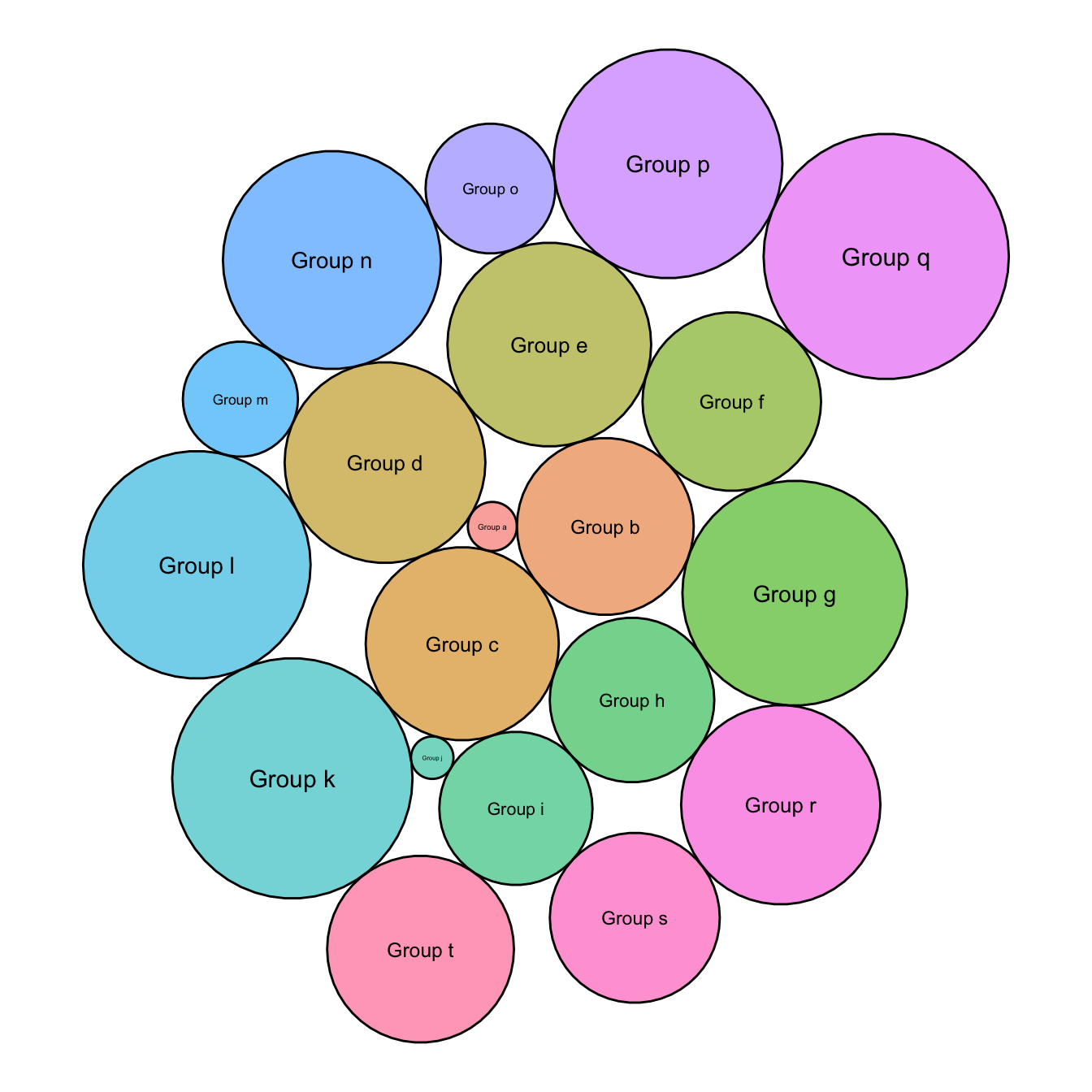# Basic circle packing with one level

This page aims to describe how to build a basic circle packing chart with only one level of hierarchy. It uses the `packcircle` package for circle position, and `ggplot2` for drawing.

This page aims to describe how to build a basic circle packing chart with only one level of hierarchy. Basically, you just represent each entity or individual of your dataset with a circle, its size depending on a provided value.

It is like a barplot, but you use circle size instead of bar length. It is close to a bubble plot, but X and Y positions do not mean anything. It is a circle version of a treemap.

Calculating the arrangement of dots is not a trivial problem. The `packcircles` library solves it and output coordinates of every points of the circle edges.

Finally, ggplot2 allows to draw shapes thanks to `geom_polygon()`.``````# Libraries
library(packcircles)
library(ggplot2)

# Create data
data <- data.frame(group=paste("Group", letters[1:20]), value=sample(seq(1,100),20))

# Generate the layout. This function return a dataframe with one line per bubble.
# It gives its center (x and y) and its radius, proportional of the value
packing <- circleProgressiveLayout(data\$value, sizetype='area')

# We can add these packing information to the initial data frame
data <- cbind(data, packing)

# Check that radius is proportional to value. We don't want a linear relationship, since it is the AREA that must be proportionnal to the value

# The next step is to go from one center + a radius to the coordinates of a circle that
# is drawn by a multitude of straight lines.
dat.gg <- circleLayoutVertices(packing, npoints=50)

# Make the plot
ggplot() +

# Make the bubbles
geom_polygon(data = dat.gg, aes(x, y, group = id, fill=as.factor(id)), colour = "black", alpha = 0.6) +

# Add text in the center of each bubble + control its size
geom_text(data = data, aes(x, y, size=value, label = group)) +
scale_size_continuous(range = c(1,4)) +

# General theme:
theme_void() +
theme(legend.position="none") +
coord_equal()``````

Related chart types

## Contact

This document is a work by Yan Holtz. Any feedback is highly encouraged. You can fill an issue on Github, drop me a message on Twitter, or send an email pasting yan.holtz.data with gmail.com.